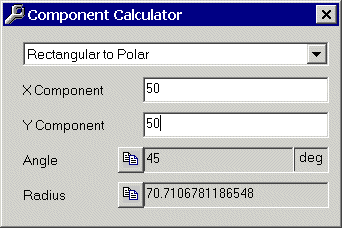Polar And Rectangular Converter

The image shown is the rectangular-to-polar calculator, which allows the user to do any of the following functions:

• provide rectangular coordinates and calculate polar
• provide polar coordinates and calculate rectangular
• provide X and R, to calculate Y and A
• provide X and A to calculate Y and R
• provide Y and R, to calculate X and A
• provide Y and A to calculate X and RMath tools included in the Mega Toolbox are:
• Least Common Multiple and Greatest Common Factor calculator
• Base converter
• Happy Number Calculator
• Polar and Rectangular Calculator
• Prime Factorization Calculator